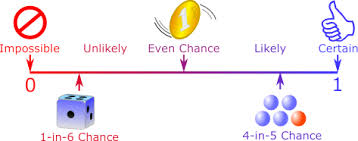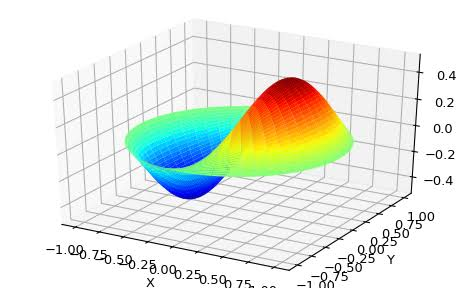#### Linear programming

This course covers linear programming example, convexity, hyperplane, simplex method, duality theory, parametric programming, Transportation problem, Assignment problem,Integer programming problem.#### Field theory

This course covers Fields, examples, characteristic of a field, subfield and prime field of a field, field extension, the degree of a field extension, algebraic extentions and transcendental extension, Adjunction of roots, splitting fields, finite fields, existence of algebraic closure, algebraically closed fields. Separable, normal and purely inseparable extensions. Perfect fields, primitive elements. Langrange’s theorem on primitive elements.Galois extensions, the fundamental theorem of Galois theory, Cyclotomic extensions, and Cyclic extensions, Applications of cyclotomic extensions and Galois theory to the constructability of regular polygons, Solvability of polynomials by radicals.#### PROBABILITY AND MATHEMATICAL STATISTICS 1

Probability theory, a branch of mathematics concerned with the analysis of random phenomena. Statistics is a tool for seeking a probabilistic description of real systems based on observing them and testing them.#### Special Functions

This course covers Hypergeometric functions, Legendre Functions,Bessel Functions,The functions of Hermite and Laguerre